Disclaimer: This is an example of a student written essay.

Any scientific information contained within this essay should not be treated as fact, this content is to be used for educational purposes only and may contain factual inaccuracies or be out of date.

# Luminescence in Low-dimensional Nanostructures

 ✅ Paper Type: Free Essay ✅ Subject: Physics ✅ Wordcount: 2748 words ✅ Published: 9th Mar 2018

• NANO AU RSY

Luminescence in Low-dimensional Nanostructures: Quantum Confinement Effect, Surface Effect

Whenever the carrier localization, at least in one spatial direction, becomes comparable or smaller than the de Broglie wavelength of carriers, quantum mechanical effects occur. In this limit the optical and electronic properties of the material change as a function of the size and the system is called a nanostructure. As the size is reduced the electronic states are shifted toward higher energy and the oscillator strength is concentrated into few transitions. Nanostructures are classified by the number of dimensions in which the carriers are confined or, alternatively, free to move. In case of confinement in only one spatial direction, the nanostructure is named a quantum well (QW). The carrier motion is frozen in one dimension but electrons and holes can still freely move over the other two directions. Therefore the QW is a quasi two-dimensional (2D) system. A structure which provides carrier confinement in two directions, allowing the motion along the remaining dimension, is called quantum wire (QWR) and it is a quasi 1D system. In the case of confinement in all three spatial coordinates, the nanostructure is denominated quantum dot (QD). QDs are 0D systems since the carrier motion is completely frozen. The physics of the quantum size effect relies on the Heisenberg uncertainty principle between the spatial position and kinetic momentum of a quantum particle. It is not possible to measure both the momentum and position of a particle to an arbitrary precision. The product of the standard deviation in space and momentum satisfies the uncertainty relation:

â-³x.â-³p ≥ â„/2 (1.26)

This equation means that the smaller is the carrier localization in the nanostructure, the larger is the spread in the momentum p, or, better said for semiconductor systems, in the crystal momentum â„k. The energy may still be well defined, but the momentum is not well defined. In bulk systems, for states around the edge of conduction and valence band, the dependence of the energy on the wavevector k is quadratic,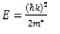Where m* is the carrier effective mass. Following this equation, the spread in the momentum â„k gives minimum kinetics energy to the localized particle. This is in contrast with the classical physics, where the lowest energy state in whatever potential corresponds to no kinetic energy. The uncertainty principle of quantum mechanics imposes a positive zero-point energy, which is approximately inversely proportional to the square of the nanostructure size. Therefore, the energy of theground state of electrons and holes in semiconductor nanostructures not only depends on the materials but also on the dimension of the confinement region.

Nanostructured materials with a size range of 1-100 nm have been the focus of recent scientific research because of their important optical properties, quantum size effects, electrical properties, chemical properties, etc. The low-dimensional materials have exhibited a wide range of optical properties that depend sensitively on both size and shape, and are of both fundamental and technological interest. The ability to control the shapes and size of nanocrystals affords an opportunity to further test theories of quantum confinement and yields materials with desirable optical characteristics from the point of view of application. The exciting emerging important application of low-dimensional nanocrystals is in light-emitting diodes (LEDs) and Displays.

If you need assistance with writing your essay, our professional essay writing service is here to help!

Recently, there has been much recent interest in low dimensional systems such as quantum well (two dimensional system), quantum wire (one dimensional system) and quantum dot (zero dimensional system). Optical properties of low-dimensional systems are substantially different from those of three-dimensional (3D) systems. The most remarkable modification comes from different distributions of energy levels and densities of states originating from the spatial confinement of electrons and holes. The simplest model for two dimensional (2D) systems is that of a particle in a box with an infinitely deep well potential, as shown in Figure 1.6. The wave functions and energy levels in the well are known from basic quantum mechanics and are described by:

Ψn(z)=(2/Lz)1/2 cos ( nπz/Lz ) (1.28 )

n = 1,2,3,…. (1.29)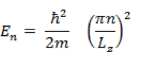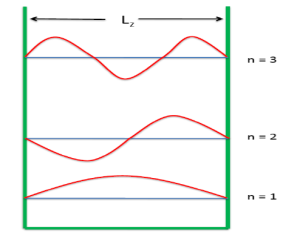Figure 1.6: A particle in a box made of infinitely tall potential barriers

In semiconductor quantum wells (two dimensional (2D) systems such as layered materials and quantum wells), both electrons and holes are confined in the same wells. The energy levels for electrons and holes are described by [1.8]:

(1.30) (1.31)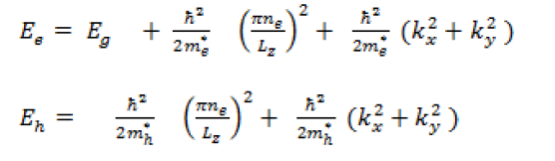Where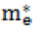and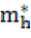are the effective masses of electron and hole, respectively

If electric dipole transitions are allowed from the valence band to the conduction band, the optical transition occurs from the state described by nh , kx , and ky to the state described by ne, kx and ky . Therefore, the optical transition takes place at energy: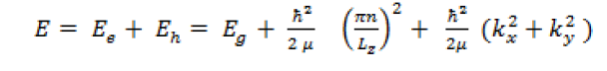(1.32)

Where μ is the reduced mass given by μ-1 =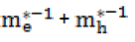The joint density of states ρ3D for the 3D for an allowed and direct transition in semiconductors is: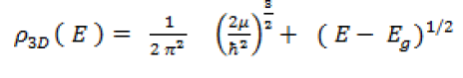(1.33)

The joint densities of states for 2D, 1D and 0D systems are: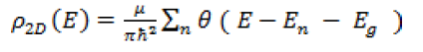(1.34)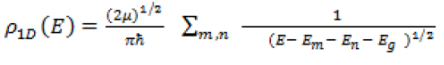(1.35)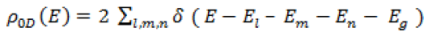(1.36)

Where θ is a step function and δ is a delta function. The sum of quantum confinement energies of electrons and holes are represented by El , Em and En ; where El , Em and En refer to the three directions of spatial confinement

Obviously the physics of the nanostructures strongly depends on their dimensionality (Figure 1.7). In a semiconductor structure a given energy usually corresponds to a large number different electronic states resulting from the carrier motion. In a bulk material where the motion can occur in three different directions the density of states increases proportionally to the square root of the energy. In quantum wells the motion in the plane gives a staircase DOS, where each step is associated with a newstate in the confining potential. In quantum wires a continuum of states is still present, but strong resonances appear in the DOS associated with the states in the confining potential. Finally in quantum dots only discrete energy states are allowed and the DOS is therefore a comb of delta functions. The possibility to concentrate the DOS in a reduced energy range is extremely important for a large variety of fundamental topics and device applications. It is at the base of the quantum Hall effect in quantum well (QW), of the quantization of the conductance in quantum wire (QWR), and of the single electron tunnelling in QDs. In the case of lasers the presence of a continuum DOS leads to losses associated with the population of states that do not contribute to the laser action. Conversely, the concentration of the DOS produces a reduction of the threshold current and enhances the thermal stability of the device operation. Clearly this property is optimized in QD structures. Due to the three-dimensional carrier confinement and the resulting discrete energy spectrum, semiconductor QDs can be regarded as artificial atoms.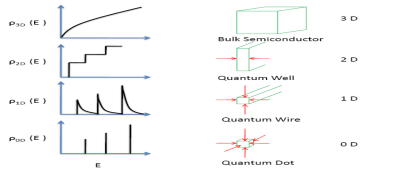Figure1. 7: Density of states of three-dimensional ( 3D ) bulk semiconductors, a two dimensional ( 2D ) quantum well, a one dimensional ( 1D ) quantum wire, and zero dimensional ( 0D ) quantum dots.

The most striking property of nanoscale semiconductor materials is the massive change in optical properties as a function of size due to quantum confinement. This is most readily manifest as a blue-shift in the absorption spectra with the decrease of the particle size. The blue-shift in the absorption spectra with decrease of particle size in semiconductor nanoparticles is due to the spatial confinement of electrons, holes, and excitons increases the kinetic energy of these particles. Simultaneously, the same spatial confinement increases the Coulomb interaction between electrons and holes. The exciton Bohr radius is a useful parameter in quantifying the quantum confinement effects in nanometer size semiconductor particles. The exciton Bohr radius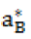is given by [1.8]: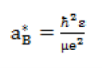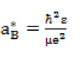(1. 37)

and an inequality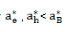holds. Here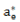and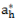are defined as: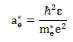and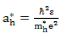(1.38 )

Where μ is the reduced mass given by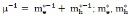are the effective masses of electron and hole, respectively. And also ε is the dielectric constant, â„ is the Planck constant.

As the particle size is reduced to approach to the exciton Bohr radius, there are drastic changes in the electronic structure and physical properties. These changes include shifts of the energy levels to higher energy, the development of discrete features in the spectra (Figure 1.8).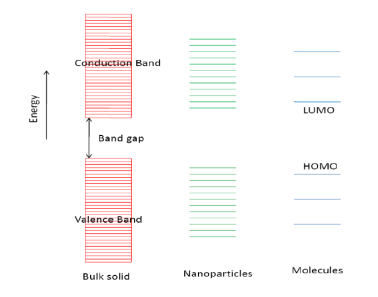Figure 1.8: A schematic models for the energy structures of bulk solids, nanoparticles and isolated molecules.

The quantum confinement effect can be classified into three categories: the weak confinement, the intermediate confinement and the strong confinement regimes, depending on the relative size of the radius of particles R compared to an electron ,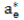a hole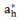, and an exciton Bohr radius ,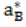respectively. In strong confinement (R < <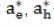, <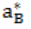), the individual motion of electrons and holes is quantized and the Coulomb int eraction energy is much smaller than the quantized kinetic energy. The ground state energy is [1.8]: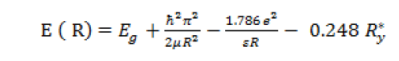(1.39)

Where the second term is the kinetic energy of electrons and holes, the third term is the Coulomb energy, and the last term is the correlation energy. In intermediate confinement (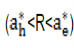), the electron motion is quantized, while the hole is bound to the electron by their Coulombic attraction. In weak confinement (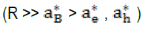), the center-of-mass motion of exciton is quantized. The ground state energy is written as: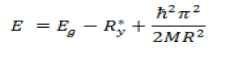(1.40 )

Where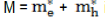is the translational mass of the exciton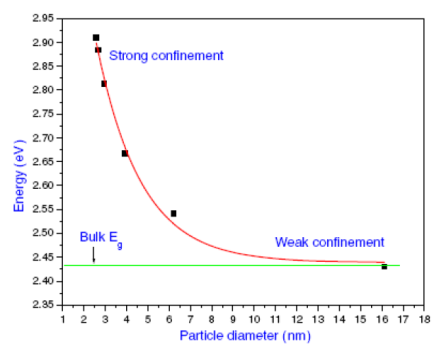Figure 1.9: Size dependence of band gap for CdS nanoparticles.

In strong confinement, there is appearance of an increase of the energy gap (blue shift of the absorption edge), which is roughly proportional to the inverse of the square of the particle radius or diameter. For example, it can be observed from Figure 1.9 that the strong confinement is exhibited by CdS particles with diameter less than ~ 6 nm (R ~ 3 nm), and this is consistent with the strong confinement effect for particles with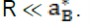The luminescence dynamics in low-dimensional nanostructures also deals with the interaction of light with the material. The interaction of light depends strongly on the surface properties of the materials. As the size of the particle approaches a few nm, both surface area to volume ratio and surface to bulk atom ratio dramatically increases. The basic relationship between the surface area to volume ratio or surface atoms to bulk atoms and the diameter of nanoparticles can be seen in Figure 1.10.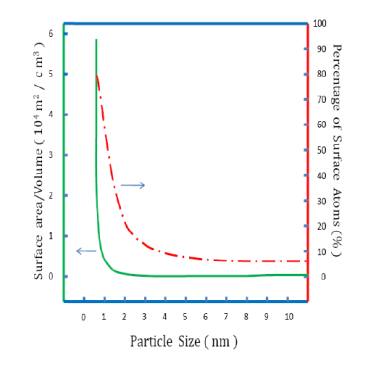Figure 1.10: Surface area to volume ratio and percentage of surface atoms (%) as a function of particle size.

It is observed that the percentage of surface atoms in corner and edge vs. Particle sizes display dramatic increase when the size is decreased below a few nm, whereas percentage of face atoms decreases. For particles of ~1 nm, more than 70% atoms are at corners or edges. This aspect is important because light interaction with material highly dependent on the atomic scale surface morphology. As in nanoparticles, a large percentage of the atoms are on or near the surface, therefore, surface states near the band gap can mix with interior levels to a substantial degree, and these effects may also influence the spacing of the energy levels. Thus in many cases it is the surface of the particles rather than the particle size that determines the optical properties. Optical excitation of semiconductor nanoparticles often leads to both band edge and deep trap luminescence. The size dependence of the excitonic or band edge emission has been studied extensively. The absence of excitonic or band edge emission has attributed to the large non-radiative decay rate of the free electrons trapped in these deeptraps of surface states. As the particle size becomes smaller, the surface to volume ratio and hence the number of surface states increases rapidly, reducing the excitonic emission. The semiconductor nanoparticles exhibit broad and Stokes-shifted luminescence arising from the deep traps of surface states [1.25 – 1.27].

View all

## DMCA / Removal Request

If you are the original writer of this essay and no longer wish to have your work published on UKEssays.com then please:

Related Services

Prices from

BHD57

Approximate costs for:

• 1000 words
• 7 day deliveryHumanity University

Dedicated to your worth and value as a human being!

Related Lectures